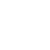Classes (extension) | UGens

# PlaneTree : UGen : AbstractFunction : ObjectExtension

Tree classifier using (hyper)planes – UGen or language-side

## Description

// Server-side (for use in synths):

PlaneTree.kr(treebuf, in, gate)

// Language-side:

PlaneTree.classify(point, treedata)

Given a specially-formatted dataset representing a hyperplane-based binary tree classifier, this unit classifies incoming data points. It can handle any dimensionality of data (the dimensionality of the incoming data points needs to match that of the tree) and can branch to arbitrary depths including some branches being deeper than others.

- treebuf - a multichannel Buffer holding data in the special tree representation. The required data format is described near the bottom of this document.

- in - the input signal to be classified (typically a multichannel signal).

- gate - on or off. While gate>0, classification is performed; otherwise the previous value is held constant.

The return value is an integer giving a binary representation of the path through the tree. For example:

9 in binary is 1001 – the first 1 always represents the root of the tree, then the remaining digits indicate you go left-left-right.

12 in binary is 1100 - the first 1 is the root, followed by right-left-left.

1 in binary is 1 so this represents the root node.

27.asBinaryDigits gives 11011, so that's right-left-right-right.

## Examples

This simple example splits 2D data into four classes:

Note that the data points are numbered starting from 1 not 0. This is deliberate and compulsory for the numbering scheme to work correctly, but a little bit confusing - in the above you might expect the three frames to be indexed as [0,1,2] but their integer references are actually [1,2,3].

------------------------

Data format

Each node in the tree is represented by a multichannel frame in a buffer (or in the language-side representation, by an array in an array). The first node must be the root node and is where classification begins. Each node consists of data defining the hyperplane used for splitting, and then flags indicating whether the split data should recurse further to a different node or whether a numeric classification result is to be returned. More explicitly, for a D-dimensional tree, each node consists of:

[

D numbers - the offset vector, subtracted from the incoming data point

D numbers - representing the normal vector, multiplied by the incoming data point (after subtraction) for classification

0 or 1 - if 1, the "left" split is a leaf and that branch terminates

0 or 1 - if 1, the "right" split is a leaf and that branch terminates

]

For dimensionality D the data must therefore have 2D + 2 channels.

Note that each "layer" of the tree is represented in sequence. The first (numbered 1) is the root, then its two children (binary 10 and 11), then their four children. Branching doesn't have to proceed to the same depth at each branch, so for example node 1001 might not exist even if node 1010 and 1011 exist, but because the frame indices are used to retrieve the data, you would still need some blank/garbage data in the 1001 frame.

(c) 2009-2010 Dan Stowell